# Bartlett's Correction to Wilk's Lambda for MANOVA

The test statistic tests for independence between a set of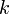$k$ numeric variables and a categorical variable containing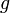$g$ groups: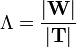$\Lambda = \frac{| \textbf{W} |}{| \textbf{T} |}$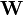$\textbf{W}$ is the withinsum of squares and cross-products matrix (computed using the Calibrated Weight),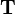$\textbf{T}$ is the total sum of squares and cross-products matrix (computed using the Calibrated Weight), and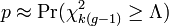$p \approx \Pr(\chi^2_{k(g-1)} \ge \Lambda)$.